### Home > INT3 > Chapter 8 > Lesson 8.3.1 > Problem8-92

8-92.

Carlos is always playing games with his graphing calculator, but now his calculator has contracted a virus. The $\boxed{\text{TRACE}}$, $\boxed{\text{ZOOM}}$, and $\boxed{\text{WINDOW}}$ functions on his calculator are not working. He needs to solve $x^{3} + 5x^{2} – 16x – 14 = 0$, so he graphs $y = x^{3} + 5x^{2} – 16x – 14$ and sees the graph below in the standard window.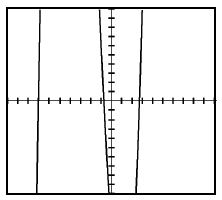1. From the graph, what number appears to be an integer solution to the equation?

$x = −7$

3. Since you know a solution to the equation, what is the factor associated with this solution?

$\left(x + 7\right)$

4. Use polynomial division to determine the other factor.

Divide $x^{3} + 5x^{2} − 16x − 14$ by $\left(x + 7\right)$ using an area model. 2 by 3 rectangle, labeled as follows: left edge, x, & 7, top edge left, x squared, interior top left, x cubed.

Labels added to the rectangle, interior top middle, negative 2, x squared, interior bottom left, 7, x squared.

Labels added to rectangle, top edge, middle, negative 2, x, interior, bottom middle, negative 14, x, interior top left, negative 2, x.

Labels added to rectangle, top edge, right, negative 2, interior bottom right, negative 14.

$x^{2} − 2x − 2$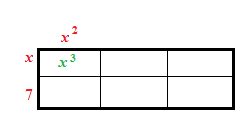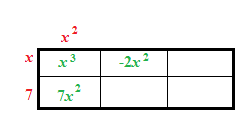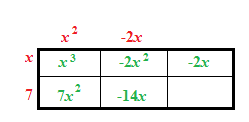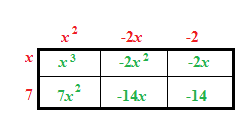5. Use your new factor to complete the following equation.

$x^{3} + 5x^{2} – 16x – 14 = 0$
$\left(x + 7\right)$(other factor)$= 0$

$\left(x + 7\right)\left(x^{2} − 2x − 2\right) = 0$

6. The “other factor” leads to two other solutions to the equation. What are the two other solutions? State all three solutions to the original equation.

Use the Quadratic Formula on the "other factor".